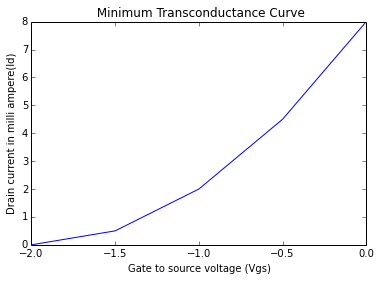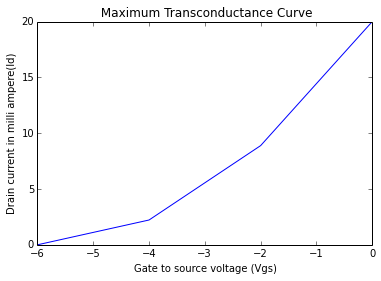# Chapter 4 : Field Effect Transistors and MOSFETs¶

## Example 4.1, Page No. 257¶

In :
#  find the drain current

import math
#variable declaration
Idss=15.0            # maximum drain current in mili-ampere
VgsOFF=-5.0          # pinch off voltage in volts
Vgs1=0.0             # gate source voltage in volts
Vgs2=-1.0            # gate source voltage in volts
Vgs3=-4.0            # gate source voltage in volts

#Calculations
Id1=Idss*(1-(Vgs1/VgsOFF))**2
Id2=Idss*(1-(Vgs2/VgsOFF))**2
Id3=Idss*(1-(Vgs3/VgsOFF))**2

#Result
print("drain current (mA) = %.f"%Id1)
print("drain current (mA) = %.1f"%Id2)
print("drain current (mA) = %.1f"%Id3)

drain current (mA) = 15
drain current (mA) = 9.6
drain current (mA) = 0.6


## Example 4.2, Page No. 257¶

In :
# find the drain current

import math
#variable declaration
Idss=12.0           # maximum drain current in mili-ampere
VgsOFF=-20.0        # pinch off voltage in volts
Vgs1=0.0            # gate source voltage in volts
Vgs2=-5.0           # gate source voltage in volts
Vgs3=-10.0          # gate source voltage in volts
Vgs4=-15.0          # gate source voltage in volts
Vgs5=-20.0          # gate source voltage in volts

#Calculations
Id1=Idss*(1-(Vgs1/VgsOFF))**2;
Id2=Idss*(1-(Vgs2/VgsOFF))**2;
Id3=Idss*(1-(Vgs3/VgsOFF))**2;
Id4=Idss*(1-(Vgs4/VgsOFF))**2;
Id5=Idss*(1-(Vgs5/VgsOFF))**2;

#Result
print("Vgs(V)\tId(mA)")
print("   %.f\t%.f"%(Vgs1,Id1))
print("  %.f \t%.f"%(Vgs2,Id2))
print(" %.f \t%.f"%(Vgs3,Id3))
print(" %.f \t%.f"%(Vgs4,Id4))
print(" %.f \t%.f"%(Vgs5,Id5))

Vgs(V)	Id(mA)
0	12
-5 	7
-10 	3
-15 	1
-20 	0


## Example 4.3.a, Page No.258¶

In :
# Plot the min transconductance curve

import math
import matplotlib.pyplot as plt
%matplotlib inline
from array import array
#variable declaration
#VGS_off=-2 to -6 volt
#IDSS=8 to 20 mA
#Formula : ID=IDSS*[1-VGS/VGS_off]^2
#Let take some values for plotting
IDSS=8.0                                       # in mA
VGS= array("f", [0, -0.5, -1.0, -1.5, -2.0])   #in Volt
VGS_off=-2        # in Volt
ID=array("f")
print(" Minimum Transcunductance Values:\n Vgs \t Id")
for i in range(0,5):
ID.append(IDSS*(1-VGS[i]/VGS_off)**2)
print("%.1f \t%.1f"%(VGS[i],ID[i]))

plt.plot(VGS,ID)
plt.title(" Minimum Transconductance Curve")
plt.xlabel("Gate to source voltage (Vgs)")
plt.ylabel("Drain current in milli ampere(Id)")
plt.show()

 Minimum Transcunductance Values:
Vgs 	 Id
0.0 	8.0
-0.5 	4.5
-1.0 	2.0
-1.5 	0.5
-2.0 	0.0## Example 4.3.b, Page No.258¶

In :
# Plot the max transconductance curve

import math
import matplotlib.pyplot as plt
%matplotlib inline
from array import array
#variable declaration
#VGS_off=-2 to -6 volt
#IDSS=8 to 20 mA
#Formula : ID=IDSS*[1-VGS/VGS_off]^2
#Let take some values for plotting
IDSS=20.0                                      # in mA
VGS= array("f", [0, -2.0, -4.0, -6.0])         #in Volt
VGS_off=-6        # in Volt
ID=array("f")
print(" Maximum Transcunductance Values:\n Vgs \t Id")
for i in range(0,4):
ID.append(IDSS*(1-VGS[i]/VGS_off)**2)
print("%.1f \t%.1f"%(VGS[i],ID[i]))

plt.plot(VGS,ID)
plt.title(" Maximum Transconductance Curve")
plt.xlabel("Gate to source voltage (Vgs)")
plt.ylabel("Drain current in milli ampere(Id)")
plt.show()
#Drain current value correspond to Vgs = -4 is wrong in the book.

 Maximum Transcunductance Values:
Vgs 	 Id
0.0 	20.0
-2.0 	8.9
-4.0 	2.2
-6.0 	0.0## Example 4.4, Page No. 261¶

In :
# drain current and transconductance

import math
#variable declaration
Idss=20.0                   # maximum drain current in mili-ampere
Vp=-8.0                     # pintc off voltage in volts
gmo=5000.0                  # in micro seconds
Vgs=-4.0                    # gate to source voltage in volts

#Calculations
Id=Idss*(1-(Vgs/Vp))**2
gm=gmo*(1-(Vgs/Vp));

#Result
print("drain current (mA) = %.f"%Id)
print("transconductance (micro-second) = %.f"%gm)

drain current (mA) = 5
transconductance (micro-second) = 2500


## Example 4.5, Page No.279¶

In :
# drain resisitance

import math
#variable declaration
Id=0.4             #drain current in mili-ampere
Vd=1.0             #drain voltage in volts
Vs=-5.0            #dc voltage in volts
Vss=-3.0           #dc voltage in volts
Vdd=5.0            #dc voltage in volts
MuCox=20.0         #in micro-ampere/volts
W=400.0            #in micro-metre
l=10.0             # in micro-metre

#Calculations
Id=((1.0/2)*(MuCox)*(W/l))
Rs=(Vss-Vs)/(Id*10**-3)
Rd=(Vdd-Vd)/(Id*10**-3)

#Result
print("The values of the resistances are as follows : ")
print("Resistance Rs is %.f K-ohm"%Rs)
print("Resistance Rd is %.f K-ohm"%Rd);

The values of the resistances are as follows :
Resistance Rs is 5 K-ohm
Resistance Rd is 10 K-ohm


## Example 4.6, Page No. 279¶

In :
# drain resisitance

import math
#variable declaration
Vdd=10.0                 # in volt
ID=0.4                   # in mA
mu_nCox=20.0             # in uA/V^2
W=100.0                  # in um
L=10.0                   # in um
Vt=2.0                   # in Volt

#Calculations
#Formula : ID=mu_n*Cox*W*(VGS-Vt)^2/(2*L)
VGS=math.sqrt(2*L*ID/(mu_nCox*10**-3*W))+(Vt)
Vd=VGS
R=(Vdd-Vd)/ID

#Result
print("Drain resistance is %.f k-ohm"%R)

Drain resistance is 15 k-ohm


## Example 4.7, Page No. 280¶

In :
# drain to source resisitance

import math
#variable declaration
Vdd=5.0                  #in volt
knwl=1.0                 #in mA/V^2
Vd=0.1                   #drain voltage
Vt=1.0                   #in Volt

#Calculations
Id=Vt*((Vdd-Vt)*Vd- (1.0/2)*0.01)   #drain current in milli ampere
Rd=(Vdd-Vd)/Id                    #resistance in killo ohms
Rds= Vd/Id                        #resistance in killo ohms

#Result
print("Effective drain to source  resistance is %.f ohm"%(Rds*1000))

Effective drain to source  resistance is 253 ohm


## Example 4.8, Page No. 280¶

In :
# Analyse the circuit

import math
#variable declaration
Vdd=10.0                 #in volt
ID=0.4                   #in mA
knwl=1.0                 #in mA/V^2
Vg=5.0                   #gate voltage in volys
Vt=1.0                   #in Volt
Rd=6.0                   #drain resistance in killo ohms
Id=0.5                   #in mA after solving the qudratic equation

#Calaculations
Vs= Id*Rd                #source voltage in volts
Vd= Vdd-Rd*Id            #drain voltage in volts
Vgs= Vg-Rd*Id            #gate to source voltage in volts

#Result
print("source voltage         = %.f V"%Vs)
print("drain voltage          = %.f V"%Vd)
print("gate to source voltage = %.f V"%Vgs)
print("drain current          = %.1f mA"%Id)
print("\nAs Vd>Vg-Vt, the transistor is operating at saturation as initially assumed.")

source voltage         = 3 V
drain voltage          = 7 V
gate to source voltage = 2 V
drain current          = 0.5 mA

As Vd>Vg-Vt, the transistor is operating at saturation as initially assumed.


## Example 4.9, Page No.281¶

In :
# drain resisitance

import math
#variable declaration
Vdd=5.0                #in volt
Id=0.5                 #in mA
knwl=1.0               #in mA/V^2
Vt=-1.0                #in Volt

#Calcualtions
#Formula : ID=mu_n*Cox*W*(VGS-Vt)^2/(2*L)
VGS=math.sqrt((2*Id/knwl))+(Vt)
Vd=3
Rd1=Vd/Id              #drain resistance in killo ohms
Vdm= Vd-Vt             #saturation mode operation
Rd2=Vdm/Id             #drain resistance in killo ohms

#Result
print("Drain resistance is %.f k-ohm"%Rd1)
print("Largest value of drain resistance maintaining saturation-region is %.f k-ohm"%Rd2)

Drain resistance is 6 k-ohm
Largest value of drain resistance maintaining saturation-region is 8 k-ohm


## Example 4.10, Page No.282¶

In :
# channel width to channel length ratio and drain resisitance

import math
#variable declaration
Id=100.0            # drain current in micro-ampere
kn=20.0             # in micro-ampere per volt^2
Vt=-1.0             # in volts
Vgs=0.0             # gate source voltage in volts
Vdd=5.0             # dc voltage in volts
Vd=1.0              # drain voltage in volts

#Calculations
wl=(2*Id/(kn*(Vgs-Vt)**2))
Rd=(Vdd-Vd)/(Id*10**-3)

#Result
print("channel width to channel ratio (W/L) = %.f"%wl)
print("drain resistance (in k-ohm)          = %.f"%Rd)
print("\nRd can vary in the range 0 to 40 k-ohm")

channel width to channel ratio (W/L) = 10
drain resistance (in k-ohm)          = 40

Rd can vary in the range 0 to 40 k-ohm


## Example 4.11, Page No. 282¶

In :
# drain to source resisitance

import math
#variable declaration
Vdd=10.0                     # in volt
knwl=1.0                     # in mA/V^2
Vd=0.1                       # drain voltage
Vt=-1.0                      # in Volt

#Calculations
Id=1*((-Vt)*Vd- (1.0/2)*0.01)# drain current in milli ampere
Rd=(Vdd-Vd)/Id               # resistance in killo ohms
Rds= Vd/Id                   # resistance in killo ohms
print("Drain to source resistance is %.f k-ohm"%Rds)

Drain to source resistance is 1 k-ohm


## Example 4.12, Page No.287¶

In :
# input resistance

import math
#variable declaration
Vdd=15.0              # in volt
knwl=0.25             # in mA/V^2
Va=50.0               # voltage
Vt=1.5                # in Volt
Id=1.06               # drain current in milli ampre
Vd= 4.4               # drain oltage in volt
Rd=10.0               # drain resistance in killo ohms
Rg=10.0               # gate resistance in killo ohms
Rl=10.0               # load resistance in killo ohms
Ii=4.3                # input current in milli ampere

#Calculations
Vgs=Vd
gm=knwl*(Vgs-Vt)      # transconductance in mA/V
ro=Va/Id              # output resistance in killo ohms
x=(Rd*Rl)/(Rd+Rl)
Av= -gm*((x*ro)/(x+ro))
Ri= Rg/Ii             # input resistance in mega ohms

print("Input resistance (Rin) = %.2f Mega-ohm"%Ri)

Input resistance (Rin) = 2.33 Mega-ohm